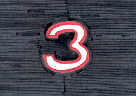# 3

This number is a prime.

Just showing those entries submitted by 'Rivera': (Click here to show all)Jason Funk © 200233 = 32 + 32 + 32. [Rivera]3 is the earliest prime p such that p^5 is the sum of 5 consecutive primes: 3^5 = 41 + 43 + 47 + 53 + 59. [Rivera]